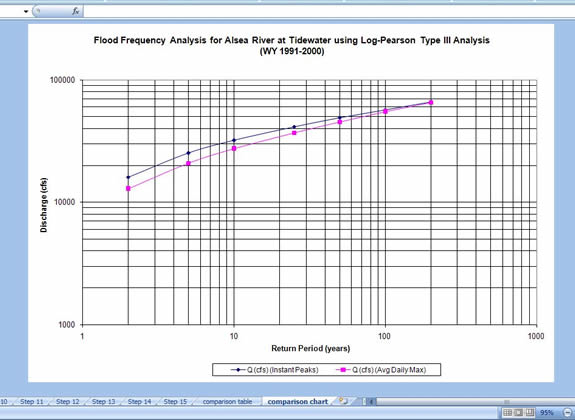# Analysis Techniques: Flood Analysis Tutorial with Daily Data (Log-Perason Type III Distribution)

## Step 1: Obtain streamflow data

• Obtain daily streamflow data from the USGS web site.
• Go to http://oregon.usgs.gov
• Select Historical Water Data
• Select Surface Water
• Select Daily Data
• Check box under Site Identifier for Site Name and Submit
• Type in Alsea under Site Name and select match any part and Submit
• Select gage at TIDEWATER (140306500)
• Select Tab-separated data
• For the tutorial, copy the data for water years 1990 through 2000 into an Excel worksheet
• Paste special as text (this will separate the data into columns
• Calculate the maximum discharge for each water year in the period of record.
• The AVERAGE, MAX, and MIN functions in excel can be used to calculate these values for each water year.  It may be more efficient to calculate the mean, maximum, and minimum flows for each water year in the period of record at one time.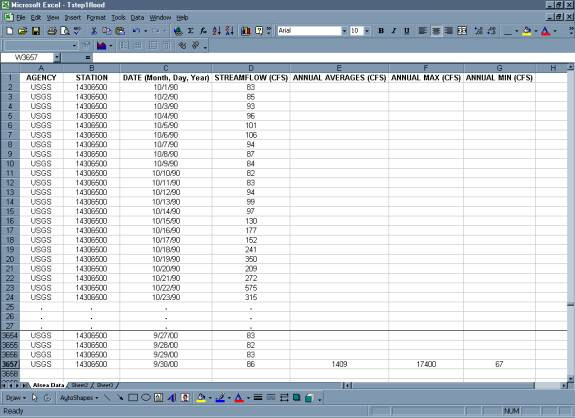## Step 2:  Organize the information in a table.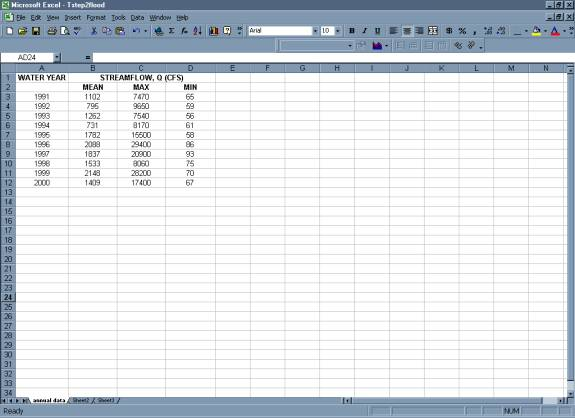## Step 3:  Rank the data from largest discharge to smallest discharge.  Add a column for Rank and number each streamflow value from 1 to n (the total number of values in your dataset).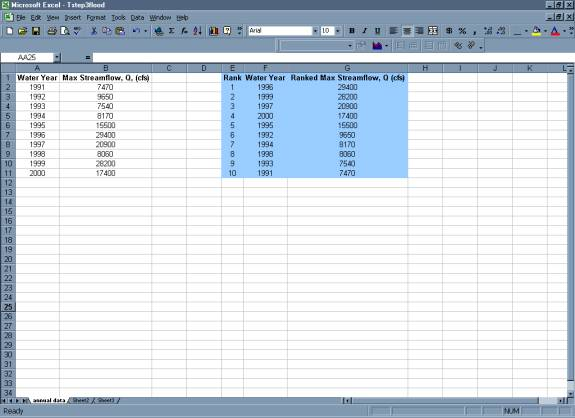## Step 4:  Create a column with the log of each max or peak streamflow using the Excel formula {log (Q)} and copy command.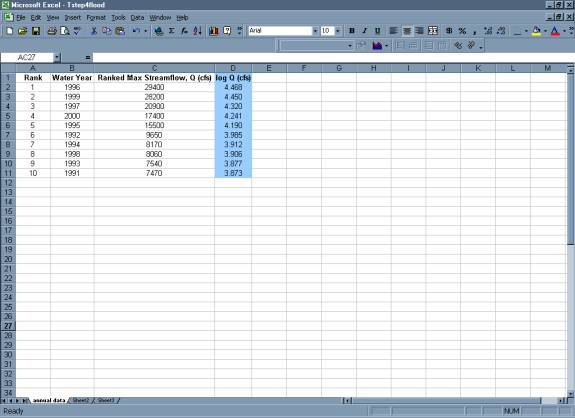## Step 5:  Calculate the Average Max Q or Peak Q and the Average of the log (Q)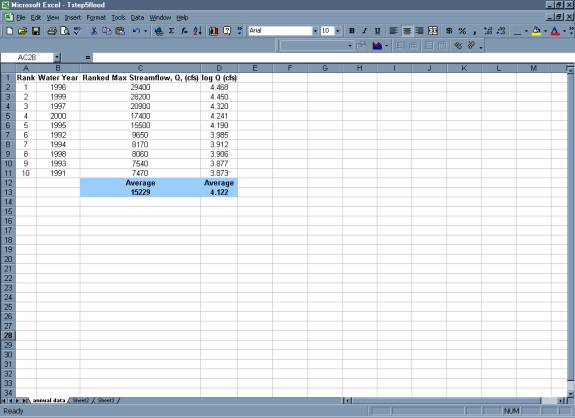## Step 6:  Create a column with the excel formula {(log Q  avg(logQ))^2}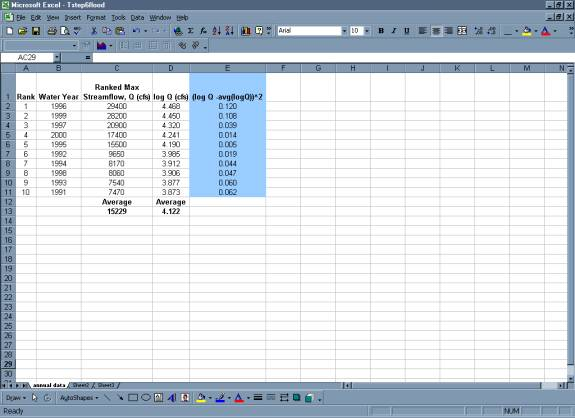## Step 7:  Create a column with the excel formula {(log Q  avg(logQ))^3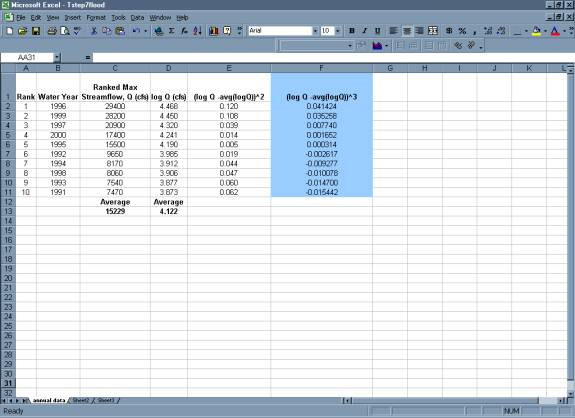## Step 8:  Create a column with the return period (Tr) for each discharge using Excel formula {(n+1)/m}.  Where n = the number of values in the dataset and m = the rank.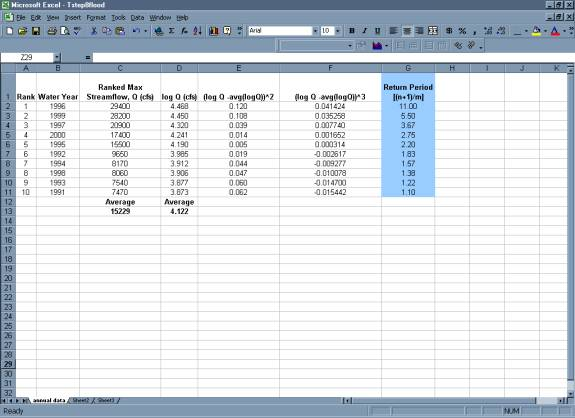## Step 9:  Complete the table with a final column showing the exceedence probability of each discharge using the excel formula {=1/Return Period or 1/Tr} and the copy command.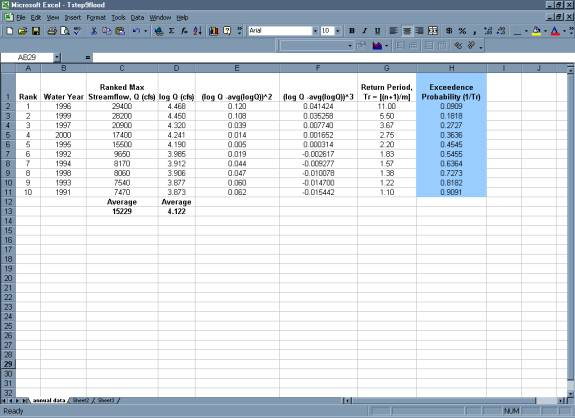## Step 10:  Calculate the Sum for the {(logQ  avg(logQ))^2} and the {(logQ  avg(logQ))^3} columns.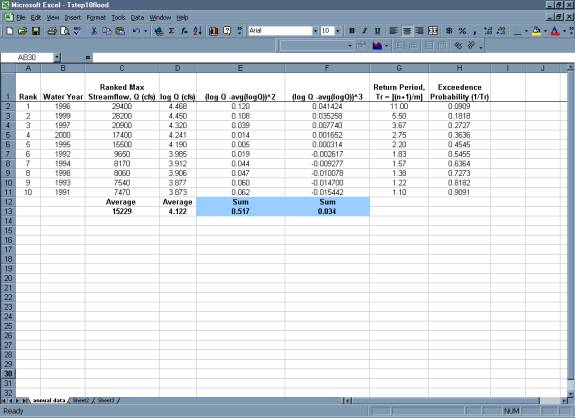## Step 11:  Calculate the variance, standard deviation, and skew coefficient as follows:

variance =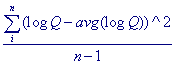standard deviation =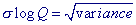skew coefficient =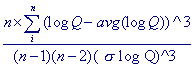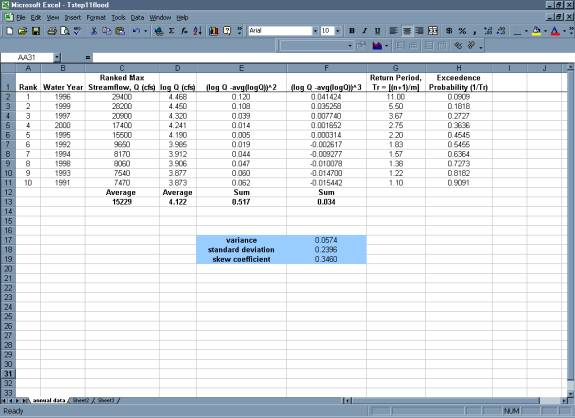## Step 12:  Calculate k values

• Use the frequency factor table and the skew coefficient to find the k values for the 2,5,10,25,50,100, and 200 recurrence intervals
• If the skew coefficient is between two given skew coefficients in the table than you can linearly extrapolate between the two numbers to get the appropriate k value. To view the frequency factor table click on the button below.

## Show Me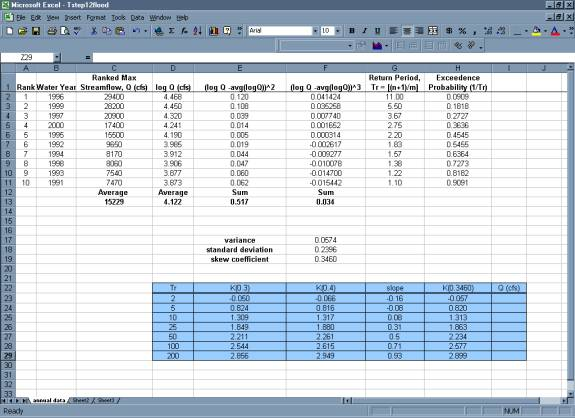## Step 13:  Using the general equation, list the discharges associated with each recurrence interval

general equation =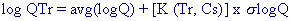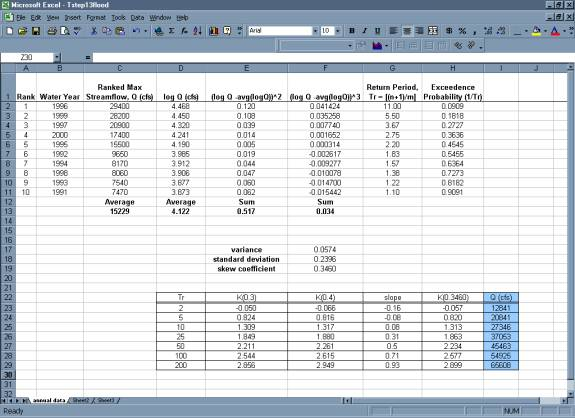## Step 14:  Create table of Discharge values found using the log  Pearson analysis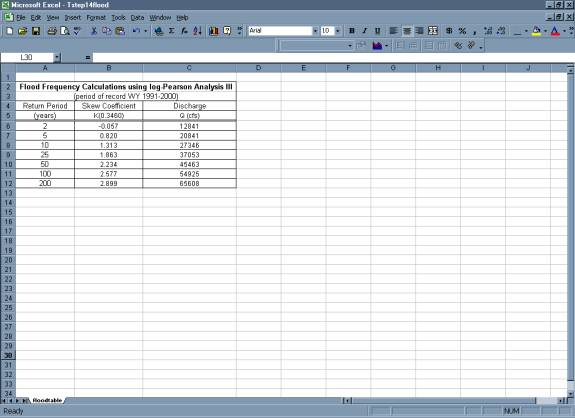## Step 15:  Create Plot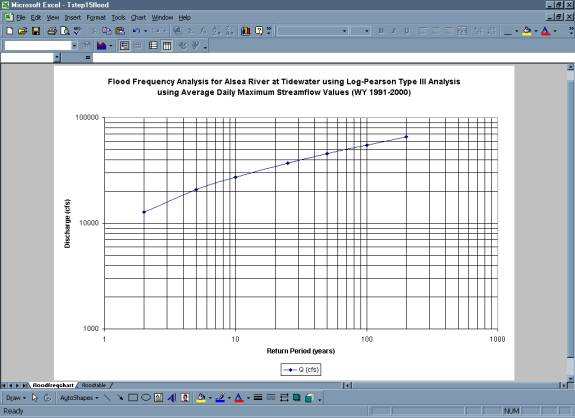• Below is a comparison of flood frequency analysis completed using mean daily data versus instantaneous discharge data. As can be seen, had you completed this analysis using instantaneous peak discharge data, the result would have been a more conservative estimation of the discharges associated with each return period.